# 概要

1. Linear Programming (LP)
2. Genetic Algorithm (GA)
3. Random Fit Placement Algorithm (RFPA)

# 介紹

VNF 置放地點的選擇可依數量分成兩種：

• 少而集中
• 硬體設備不用買那麼多
• 距離遠，傳輸成本增加
• 多而分散
• 需買較多硬體，且需計算利用率
• 距離近，傳輸成本低

# 參考架構

NFV 資源分配問題可以分成三種步驟：

1. VNFs Chain Composition (VNFs-CC)
• 在 data center 跟 mobile network 尤其重要
• IETF Network and Service Chaining Working Groups 有在貢獻
2. VFN Forwarding Graph Embedding (VNF-FGE)
• 根據需求尋找VNF 置放位置
• 計算資源
• 能源資源
• 網路負載平衡
• 也被叫做 VNF placement problem
3. NFs Scheduling ( VNFs-SCH)
• 對 VNF 的執行做排程
• 以降低整體運行時間

## SFC in Mobile Core Network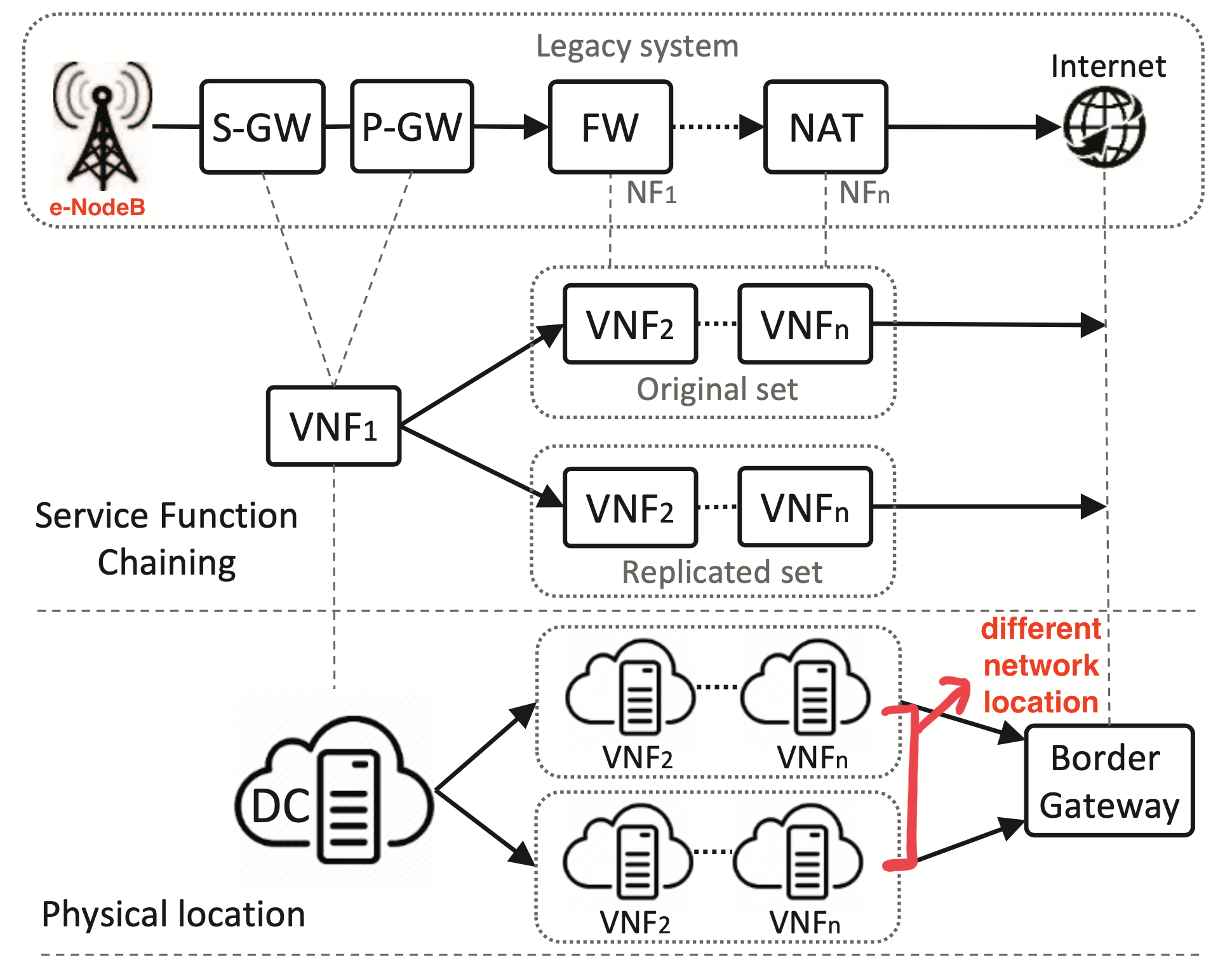S-GW, P-GW 對應到 VNF1，這兩個都是 non-replicable 的，後面的設備如防火牆與 NAT 都是 replicable。

## Network Traffic Model

1. background traffic
• 從每個核心節點（core node）產生的流量，會流到其他節點
2. data center traffic
• 從 data center 產生，座落在不同的網路部分，導向到 Internet gateway
• 由終端用戶產生，通常是 TCP
• 在接上網際網路前會經過一連串的 network function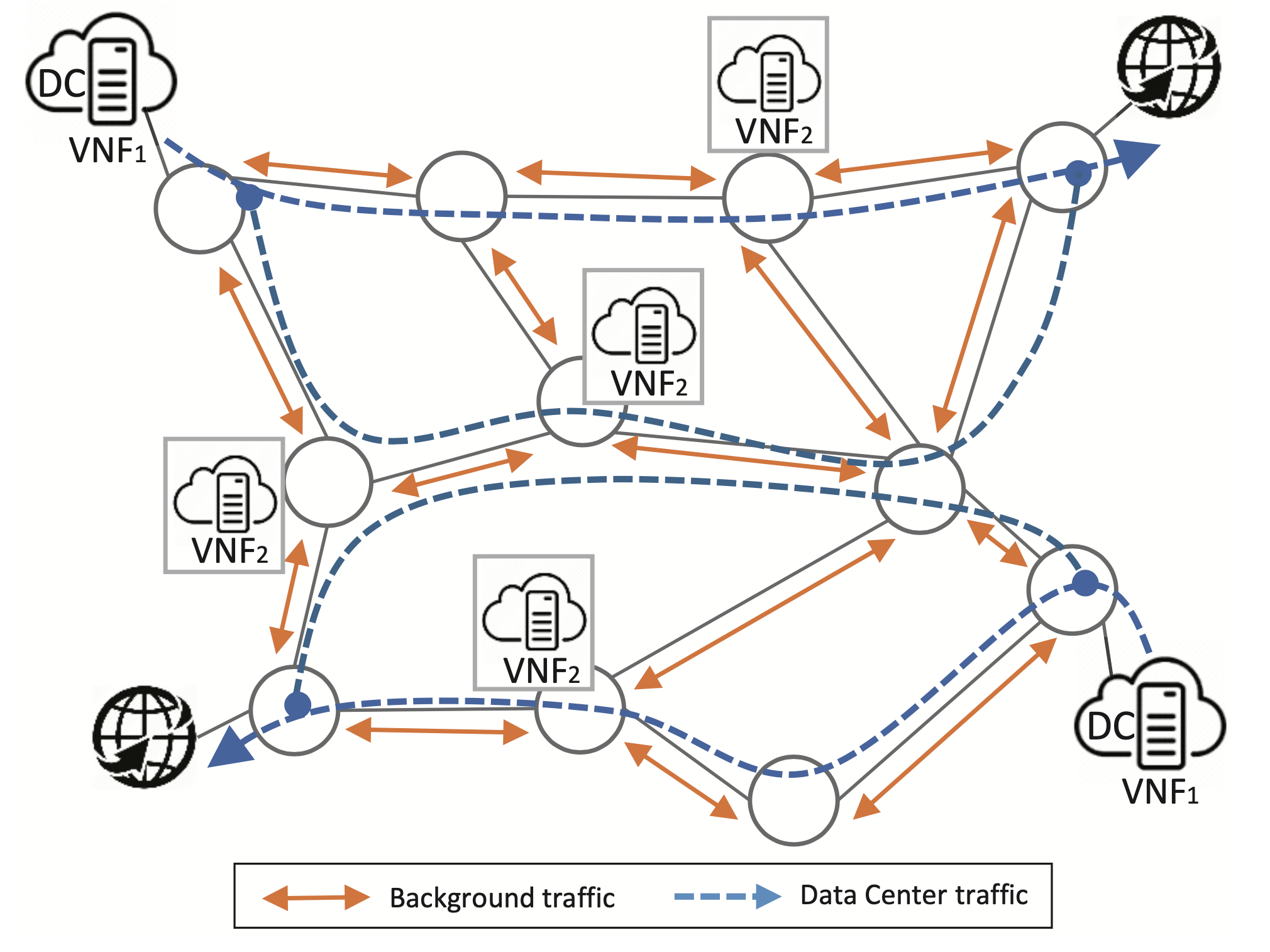# 最佳化模型

$$Minimize: \sum_{\ell \in \overrightarrow{L}}{K_\ell}$$

$$K_{\ell} \geq y_{i}\left(\sum_{s \in \vec{S}} \sum_{p \in \vec{P}} \sum_{\lambda \in \vec{\Lambda}{b g \mid s}} \frac{\lambda \cdot\left(R{p}^{\lambda} \mid R_{p, s}^{\lambda}\right) \cdot t_{p}^{\ell}}{c_{\ell}}\right)$$

• $R^{\lambda}_{p}$ 是用在 TE 的情況
• $R^{\lambda}_{p, s}$ 是用在 RA 的情況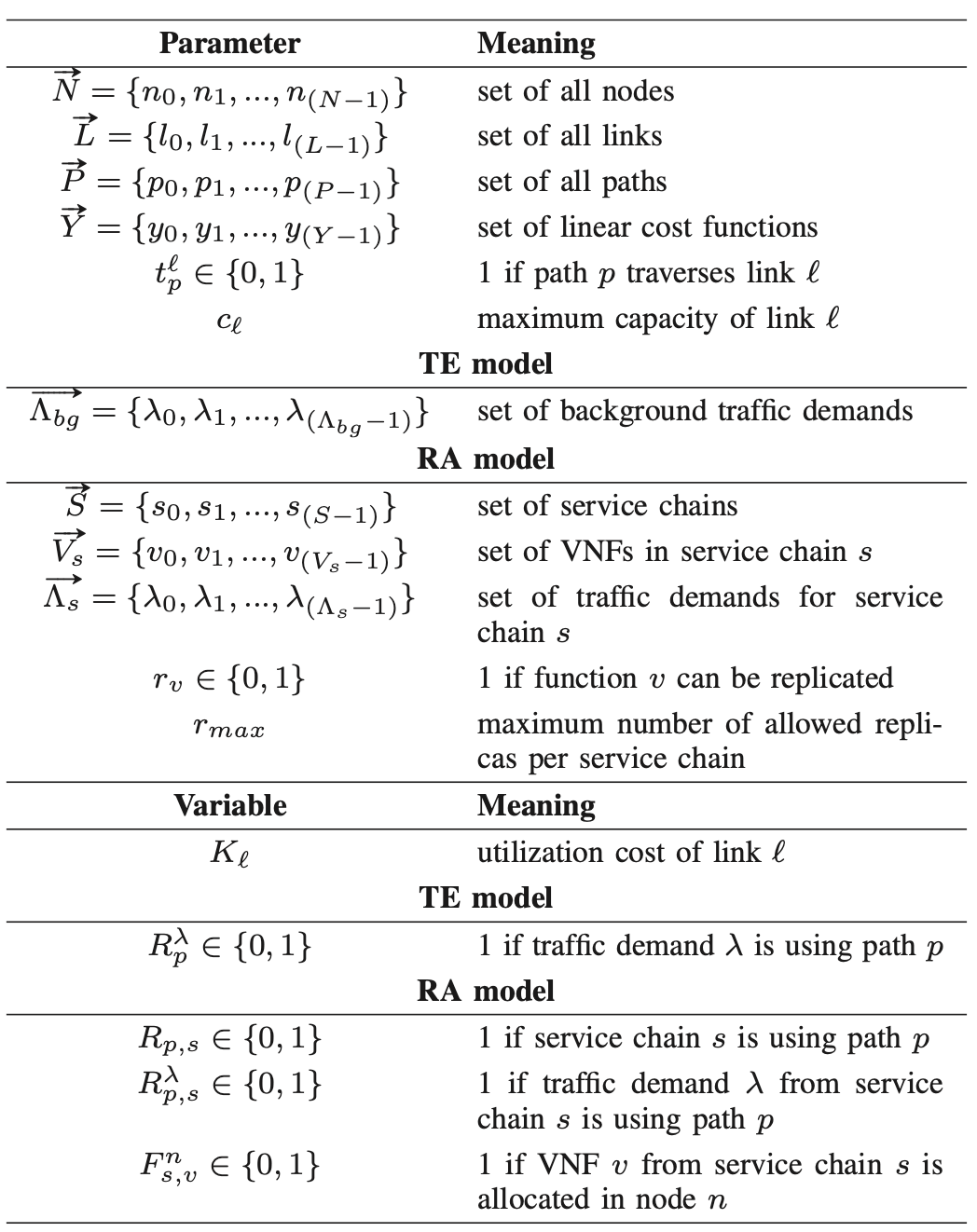Routing constrain:

for TE: $\forall \lambda \in \overrightarrow{\Lambda_{b g}}: \sum_{p \in \vec{P}} R_{p}^{\lambda}=1$

for RA: $\forall \lambda \in \vec{\Lambda_{s}}: \sum_{s \in \vec{S}} \sum_{p \in \vec{P}} R_{p, s}^{\lambda}=1$

traffic demand 只能在 service chain 使用時而存在

$$\forall p \in \vec{P}, \forall s \in \vec{S}, \forall \lambda \in \vec{\Lambda_{s}}: R_{p, s}^{\lambda} \leq R_{p, s}$$

$$\forall s \in \vec{S}: 1 \leq \sum_{p \in \vec{P}} R_{p, s} \leq r_{m a x}+1$$

SFC 中所有的 VNF 都有分配到 path 上的 node

$$\forall p \in \vec{P}, \forall s \in \vec{S}, \forall v \in \vec{V_{s}}: R_{p, s} \leq \sum_{n \in p} F_{s, v}^{n}$$

$$\begin{array}{c}\forall s \in \vec{S}, \forall p_{1} \in \vec{P}, \forall p_{2} \in \vec{P}, \forall v \in \vec{V_{s}}, \forall n \in p_{1}, p_{2}: R_{p_{1}, s}+R_{p_{2}, s}+2 F_{s, v}^{n} \cdot r_{v} \leq 3\end{array}$$

$$\left(\sum_{m=0}^{n-1} F_{s, v-1}^{m}\right)-F_{s, v}^{n} \geq R_{p, s}-1$$

$$\forall n \in \vec{N}: \sum_{s \in \vec{S}} \sum_{v \in \vec{V}{s}} F{s, v}^{n} \leq 1$$

replicable function 的數量上限

$$\sum_{n}^{N} F_{s, v}^{n} \leq 1+r_{m a x} \cdot r_{v}$$

# 啟發式演算法

VNF allocation 已經被知道是 NP-hard 問題，linear programming model 只能用在小型網路當中，大型網路必須要用啟發式演算法。

## Genetic Algorithm (Algorithm 1)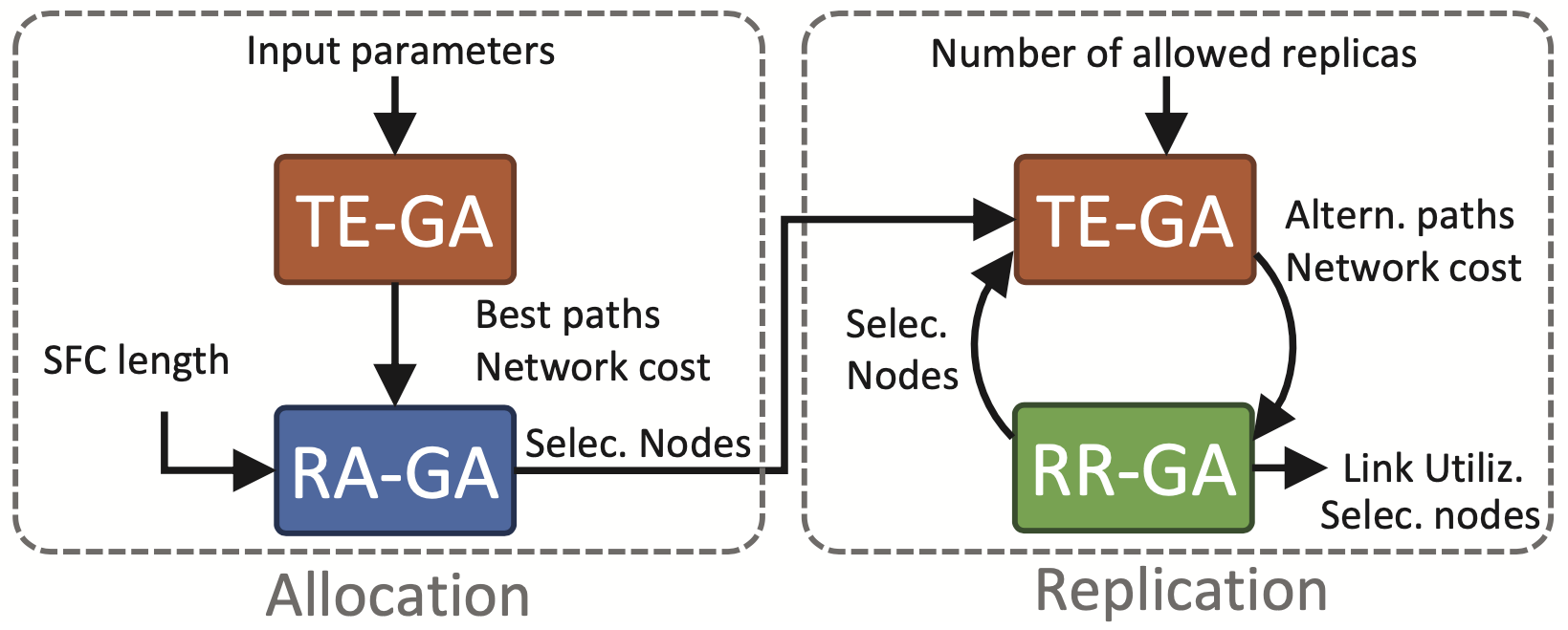1. Traffic Engineering (TE-GA)
• 從輸入參數挑選一些有資格的 path
• 計算網路開銷
• 輸出 path 將作為 RA-GA 的輸入
2. Resource Allocation (RA-GA)
• 負責 VNF 的分配
• 選擇節點
• 根據 data center 流量的網路開銷
• 根據 TE-GA 給的 path
• 選到的節點將會作為「尋找替代路徑」的參數
• 選擇的節點將作為 RR-GA 的輸入
3. Resource Replication (RR-GA)
• 負責分配 replica
• 根據上限值
• 一直分配 replica 直到所增加的 replica 無法改善 cost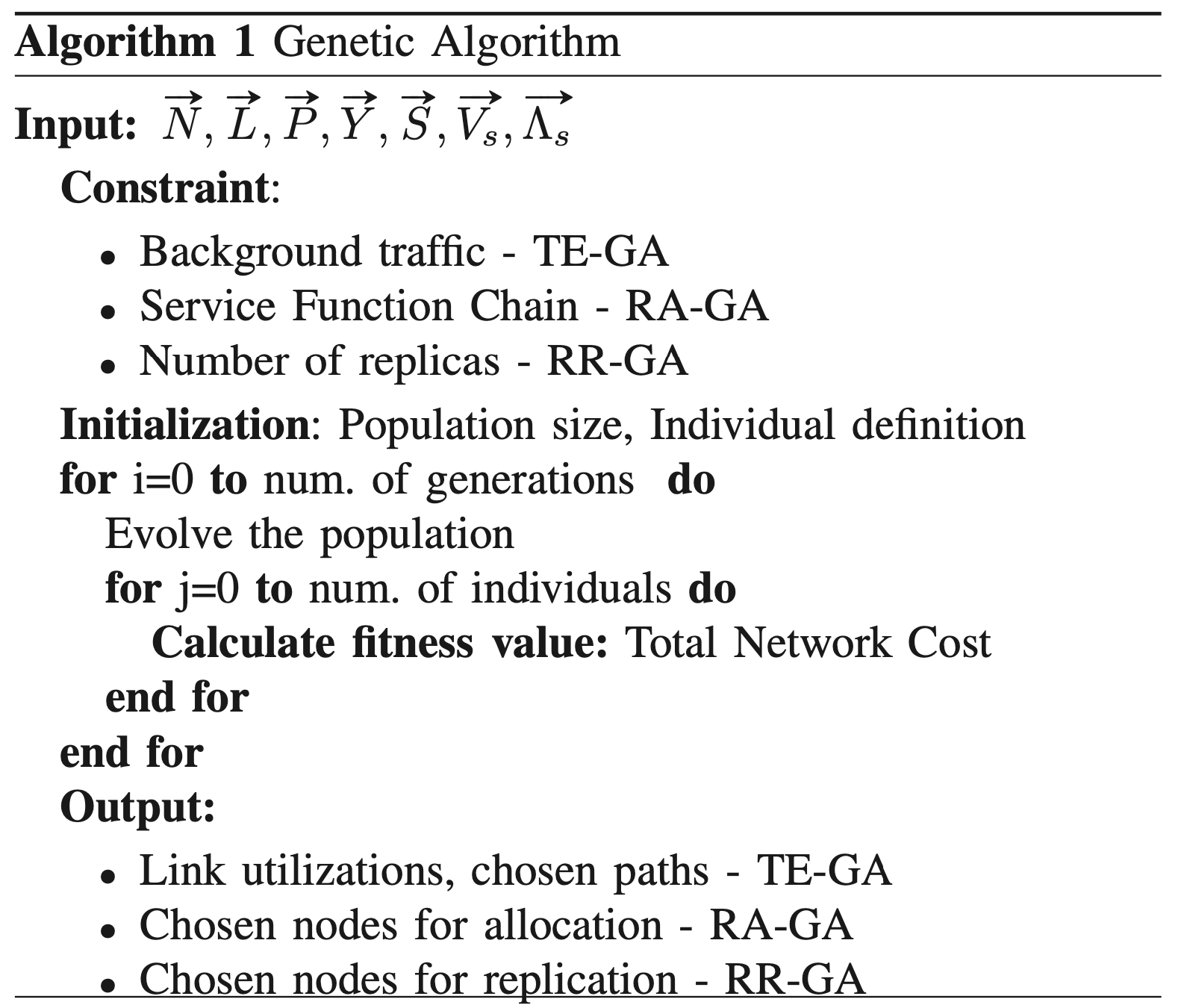## Random-Fit Placement Algorithm

• 隨機擺放 VNF
• 依照正確順序尋找可行的 path，越多 replica 越好
• 對於每個 traffic deman，只會隨機選擇一條 path
• 輸出結果會是 total network cost、被選擇的節點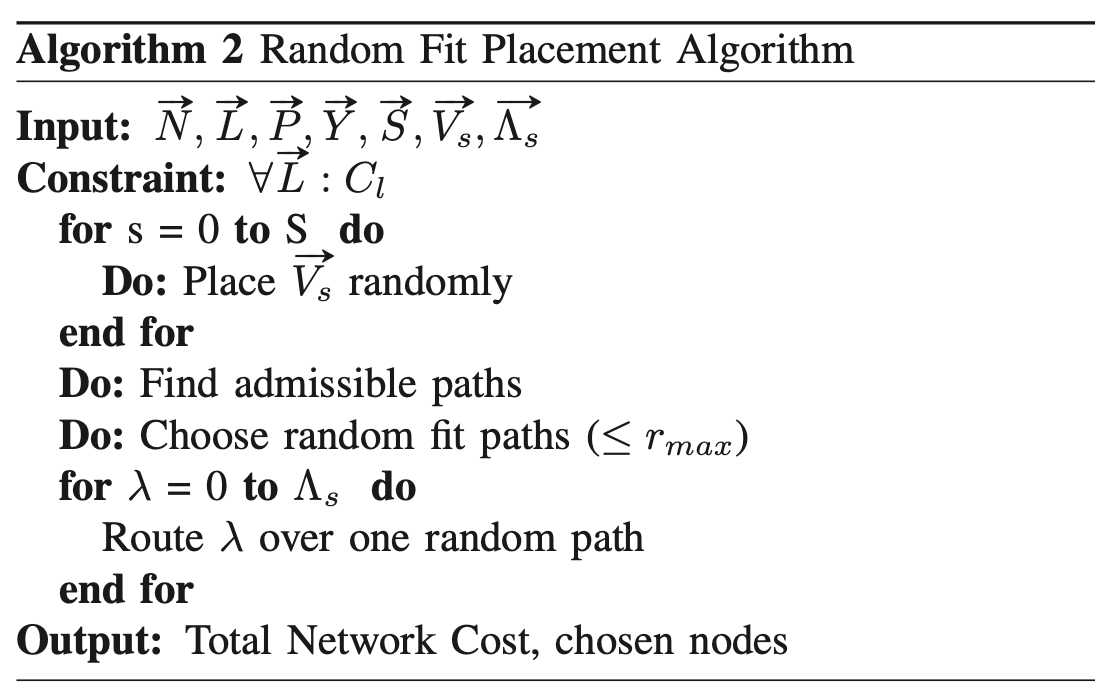# 效能評估

1. Genetic Algorithm (作者提出的）
2. Gurobi Optimizer (Linear Programming solver)
3. Random Allocation Approach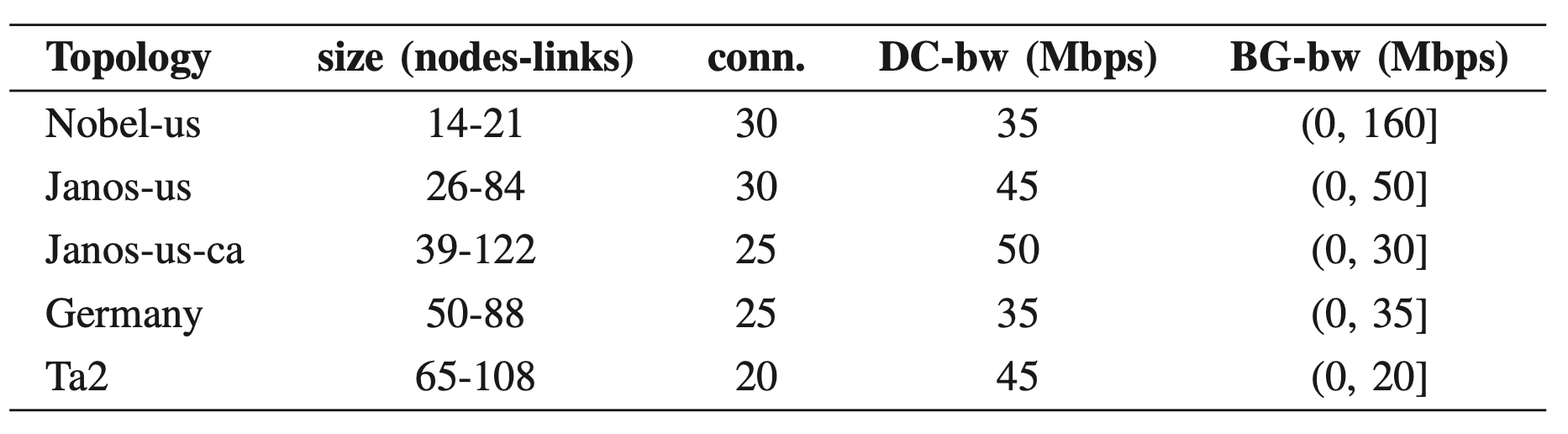Background traffic 隨機產生，間隔為 background bandwidth，並且確保 TE model 的開銷永遠小於 1，也就是不會有瓶頸的產生。

Service chain 的長度為兩個 end-point (例如 S-GW, P-GW datacenter & border gateway)、與一個位於中間的 VNF。

Nobel-us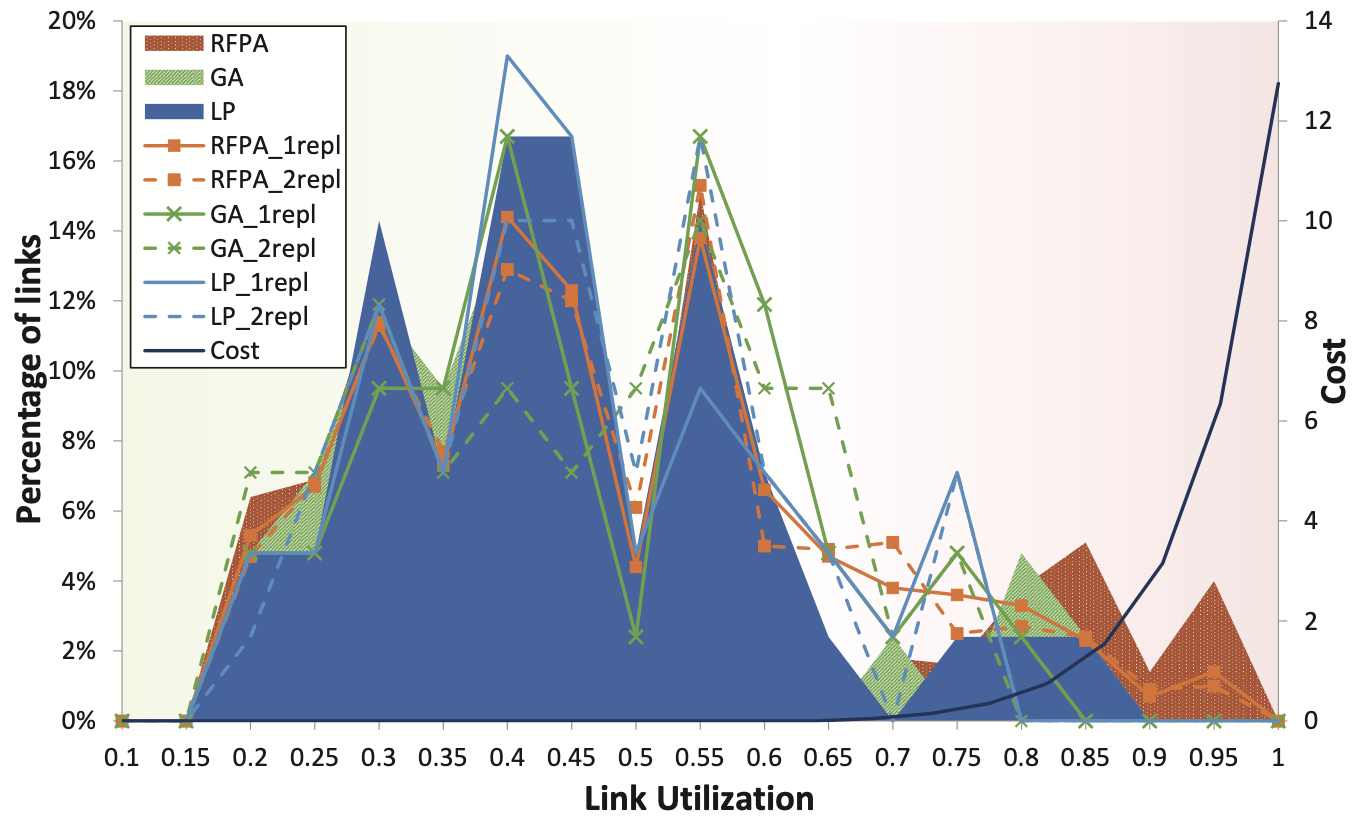Janos-us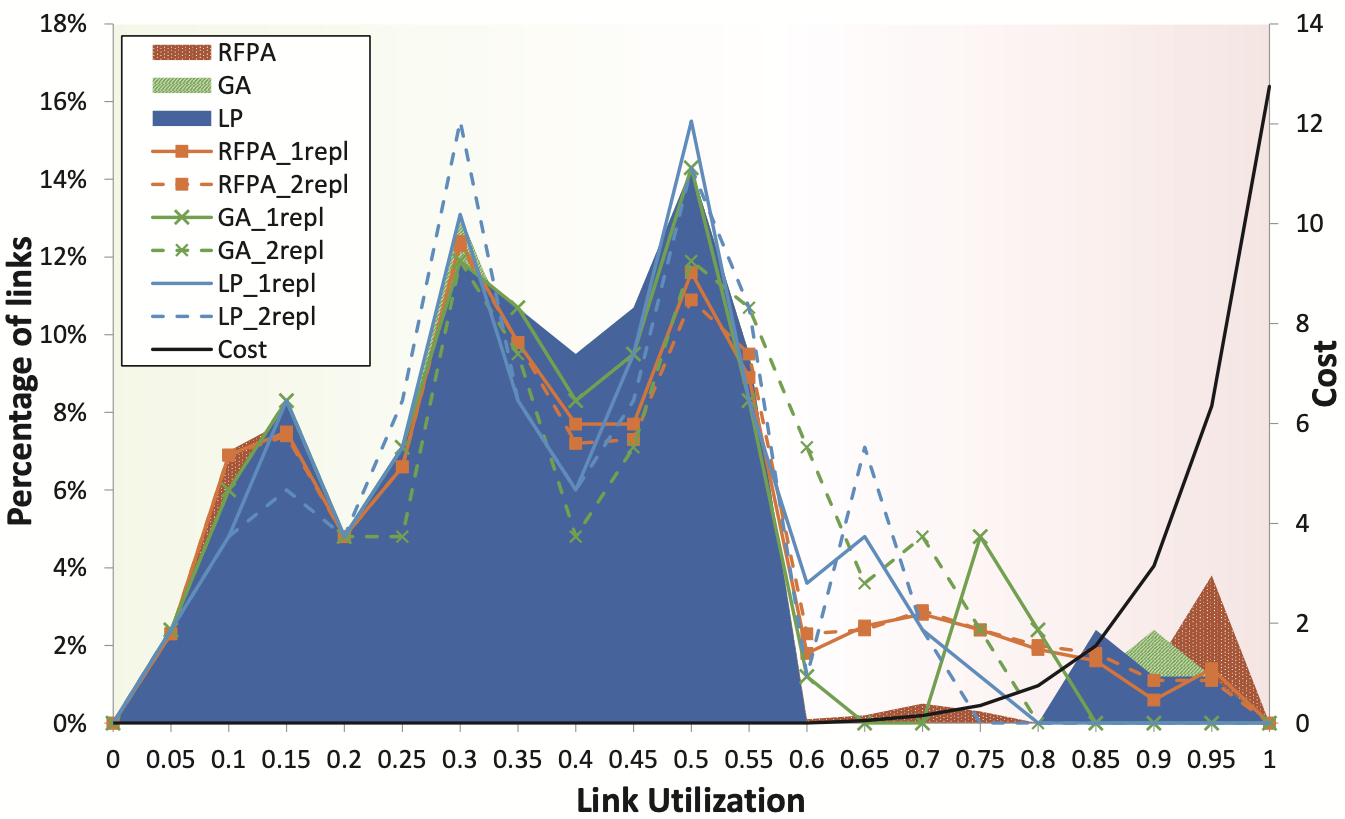## Network cost & # of replicas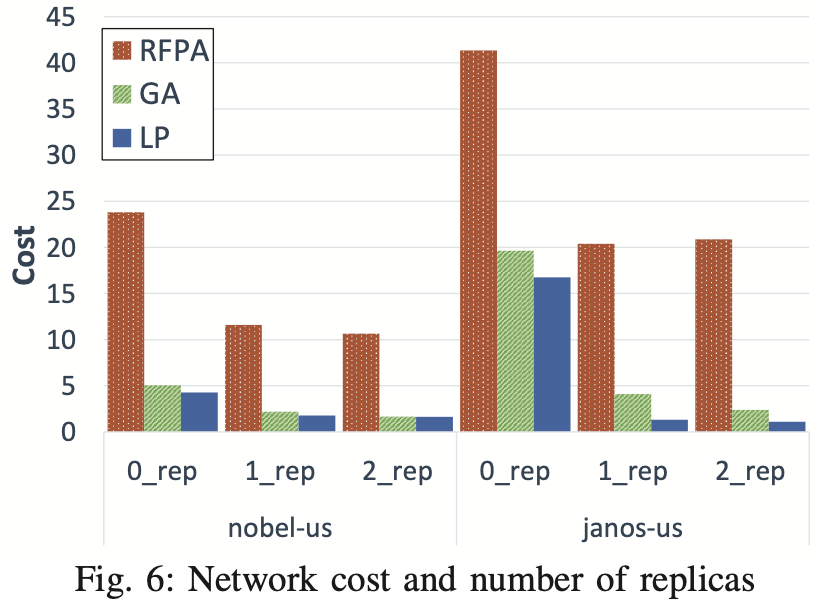## Computation Time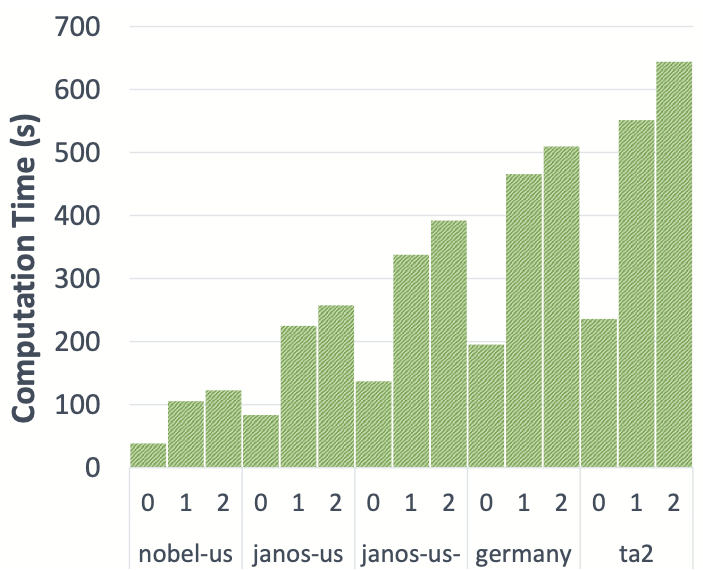# 名詞解釋

## 整數規劃 (Integer Programming)

### 整數規劃的種類

1. 純整數規劃：所有決策變數均要求為整數的整數規劃
2. 混合整數規劃 (Mixed Integer Programming, MIP)：部分決策變數均要求為整數的整數規劃
3. 純0－1整數規劃：所有決策變數均要求為0－1的整數規劃
4. 混合0－1規劃：部分決策變數均要求為0－1的整數規劃

## SAE 結構體系

System Architecture Evolution 是 3GPP 所制定的 LTE 無線通信核心網路標準，傳輸全使用 IP 網路，從而支援系統的 control plane 與 data plane 分離。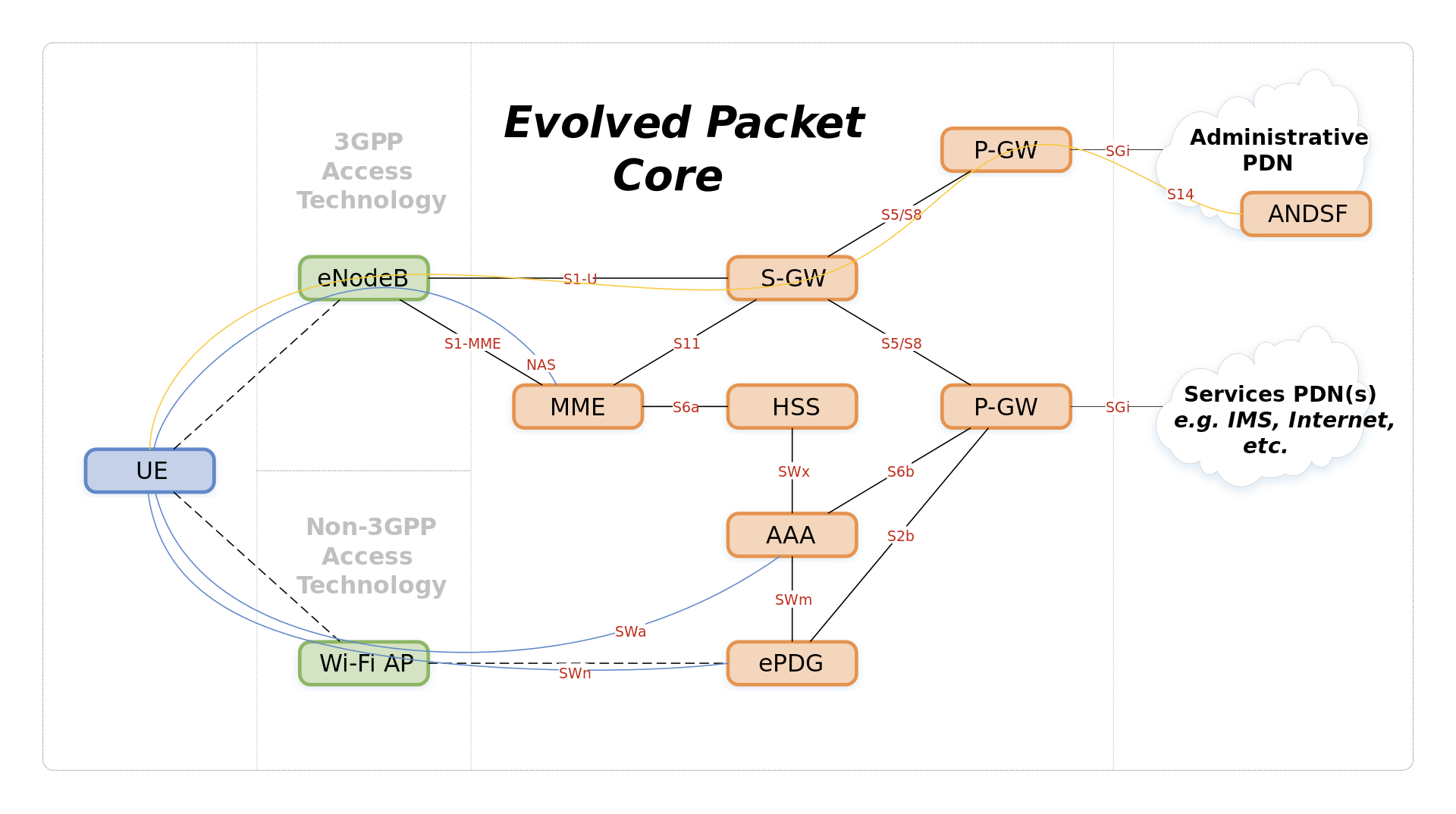### P-GW

PDN 閘道器（PGW，PDN Gateway）：PDN 閘道器作為連線點， 為 UE 提供與 公共資料網 （PDN）之間的傳輸 。一個 UE 可以同時透過多個 PGW 訪問多個 PDN。PGW 實現控制策略的實施、針對使用者的資料包過濾、計費、 合法監聽 與資料包篩選。 PGW 的另一個關鍵作用的是作為 3GPP 和非 3GPP 網路（例如 WiMAX 和 3GPP2 的 CDMA 1X 和 EvDO）之間的移動性管理錨點。

## SNDLib

### What is SNDlib?

SNDlib is a library of test instances for Survivable fixed telecommunication Network Design. Its purpose is

• to make realistic network design test instances available to the research community,
• to serve as a standardized benchmark for testing, evaluating, and comparing network design models and algorithms,
• to be a source of information and resources related to fixed network design, and
• to provide a contact platform for researchers and practitioners working in this field.

### Why SNDlib?

In the field of telecommunication network design problems, proposed solution approaches range from LP-/IP-based models and algorithms such as branch-and-bound, row and column generation, or Lagrangian relaxation to meta-heuristics such as evolutionary algorithms, simulated annealing and tabu search.